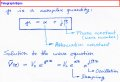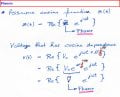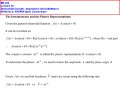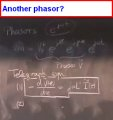# Phasors + Wave equation

#### Arausio

Joined Jun 18, 2015
41
Hello guys, I need some of your expertise to help me understand the notion of phasors and their representation in the wave equation. From school the notes show that a phasor is $$V_{0}e^{\alpha}$$ and this is shown in the page marked phasors below. However, reading from wikipedia a phasor for a harmonic signal such as $$a(t)=Acos(\omega t + \phi)$$ is
$$Re\left \{ b(t) \right \}$$ where $$b(t)$$ is an imaginary number,
$$b(t)=Ae^{\mathit{j (\omega t+ \phi)}}=Ae^{\mathit{j \phi}}e^{\mathit{j \omega t}}$$. Now, the real part of $$\widehat{A}=Ae^{\textit{j} \phi}$$ multiplied by $$cos(\omega t)$$ gives us the original $$a(t)$$ back. I.e. $$Re\left \{ \widehat{A} \right \}=Acos(\phi); a(t)=Acos(\phi)*cos(\omega t)$$
Moreover, looking at the KFUPM Open Courseware I also found that a phasor should have a j multiplied in the exponent. So, what is the proper representation? Whom should I believe and what exactly should I leave in my notes?#### Papabravo

Joined Feb 24, 2006
20,383
Phasors are a convenient way to represent periodic functions of time such as AC voltage and current. They represent real things that you can observe and measure, but they use algebra of complex numbers, to keep track of things like magnitude and phase.

Point #1: A complex number can be represented in multiple ways:
1. In Cartesian Coordinates as a + jb, where a and b are real numbers an j is the imaginary unit aka √(-1).
2. In Polar Coordinates as a magnitude and a phase, for example 6.3 ∠ 30°. In this case the magnitude is 6.3 and the phase angle is 30°
Point #2: You can easily convert a Cartesian Form to a Polar Form
1. If we have √3 + j in Cartesian Coordinates, this is equivalent to 2 ∠ 30°
2. If we have 2 ∠ 60° in Polar Coordinates, this is equivalent to 1 + j√3
Point #3: A phasor is a representation of a periodic sinewave that has a magnitude and a phase. It is a function of time, but time is not shown explicitly in the representation. For purposes of calculation the algebra of phasors does not require the explicit use of time t.

Point #4: A complex exponential may also be used to represent a periodic sinewave due to the following identity:

$$e ^ {j \phi} = cos(\phi)+j sin(\phi)$$

Point #5: The explicit time dependence is encapsulated in the following term:

$$e ^ {j \omega t} = cos(\omega t) + j sin(\omega t)$$

This is a unit vector the rotates around the origin in a counter clockwise direction.

If it helps you to visualize what is going on, you can think of $$A e^{j \phi}$$ as the initial position of the phasor at time t = 0. Also the magnitude of $$e ^ {j \omega t}$$ is 1 and the initial angle is 0° at t = 0.

In summary:
1. a + jb can be treated as a phasor
2. M ∠ ∅ can be treated as a phasor
3. $$A e^{j \phi}e^{j \omega t}$$ can be treated as a phasor
Here is a reference for Phasor Algebra

https://en.wikiversity.org/wiki/Phasor_algebra

Last edited:
•Arausio

#### Arausio

Joined Jun 18, 2015
41
Papabravo,
Thank you for your post it helped me a lot! Now, what I'm thinking is trying to decode the professor's trail of thought going from phasors to the wave equation. So, on his phasors page he represented phasor without the j which is incorrect (from what I understood). However, in regards to $$\widetilde{V}(z)=V_{0}e^{\gamma z}=V_{0}e^{\alpha z}e^{\textit{j}\beta z}$$ where $$\gamma=\alpha +\textit{j} \beta$$(phasor) the $$V_{0}e^{\gamma z}$$ represents only the phasor component where the exponential looks like $$V_{0}e^{\textit{j} \phi}=V_{0}e^{\alpha z}e^{\textit{j}\beta z}$$ after plugging in for gamma. Sorry if I'm asking easy questions it's just I'm still a little confused on the components in the wave equation which constitute the attenuation and oscillating constants and what represents the phasor in it.

#### Papabravo

Joined Feb 24, 2006
20,383
The difference is fairly subtle. Any exponential terms without a j in them, can be thought of as contributing to the magnitude rather than the phase . In the simple case of a periodic waveform you have only the phase angle changing with time. In the more general case you have both the magnitude and the phase changing with time. In your equation replace z with t and you have:

$$V_0 e^{\gamma t}=V_0 e^{\alpha t} e^{j \beta t}$$

Now using the alternative phasor notation in polar form:
1. The magnitude is $$V_0 e^ {\alpha t}$$. The magnitude starts at V0 and α must be strictly ≤ 0 or it will diverge to ∞. With α < 0 you have a damped response.
2. The remaining term shows the explicit time dependence of the phase angle.
Let me sum up:
1. With α < 0, you have a damped sinusoidal response.
2. With α = 0, you have periodic solution at a single frequency determined by β.
3. With α > 0, you have a divergent solution which goes off to ∞.
Got it?

Extra Credit: Examine the derivative of a phasor, substitute it back into the original equation, and see if it makes sense.

Last edited:
•Arausio

#### WBahn

Joined Mar 31, 2012
29,156
There are several waves of linking a phasor to a sinusoidal signal and all that matters is that you are consistent in how you do it.

The key relationship is

$$Ae^{j \( \omega t+\phi$$} \; = \; A\cos(\omega t+\phi) \, + \, j \, A\sin(\omega t+\phi)
\)

The two most common ways mapping the phasor to the sinusoidal signal are to exploit one of the following relationships:

$$A\cos(\omega t+\phi) \; = \; Re \{ Ae^{j \( \omega t+\phi$$} \} \; = \; Re \{ Ae^{j \phi}e^{j \omega t} \}
\)
or
$$A\sin(\omega t+\phi) \; = \; Im \{ Ae^{j \( \omega t+\phi$$} \} \; = \; Im \{ Ae^{j \phi}e^{j \omega t} \}
\)

Since the $$e^{j \omega t}$$ is a multiplying term that does not change, we essentially factor it out of all of our work and work with just the $$Ae^{j \phi}$$ as our "phasor".

The "phasor" is just a convenient way of keeping track of the magnitude (A) and the phase (φ) of the complex exponential. This is because if you add, subtract, multiply, or divide complex exponentials, the magnitude and phase combine the same way that the components of the phasors do.

Last edited:

#### Papabravo

Joined Feb 24, 2006
20,383
There are several waves of linking a phasor to a sinusoidal signal and all that matters is that you are consistent in how you do it.

The key relationship is

$$Ae^{j \omega t} \; = \; A\cos(\omega t) \, + \, j \, A\sin(\omega t)$$

The two most common ways mapping the phasor to the sinusoidal signal are to exploit one of the following relationships:

$$A\cos(\omega t) \; = \; Re \{ Ae^{j \omega t} \}$$
or
$$A\sin(\omega t) \; = \; Im \{ Ae^{j \omega t} \}$$

The "phasor" is just a convenient way of keeping track of the magnitude (A) and the phase (ωt) of the complex exponential. This is because if you add, subtract, multiply, or divide complex exponentials, the magnitude and phase combine the same way that the components of the phasors do.
Yup! What he said.

•Arausio

#### Arausio

Joined Jun 18, 2015
41
Hello guys, thank you for the help. I have another phasor representation straight from the board. It seems a bit incorrect to me... since $$\gamma = \alpha +\textit{j} \beta$$
$$V(t)=V_{0}^{+}e^{\textit{j}\phi }e^{\textit{j}\gamma t}e^{\textit{j}\omega t}$$ where the phasor component is $$V_{0}^{+}e^{\textit{j}\phi }e^{\textit{j}\gamma t}$$
Anyway, what I understood from all this is the following (please do correct me if I'm wrong):
1) Phasor consists of a complex amplitude + phase angle
2) The wave eqn. $$\widetilde{V}(z)=V_{0}e^{\gamma z}=V_{0}e^{\alpha z}e^{\textit{j}\beta z}$$ is the phasor where the frequency component $$\omega z$$ of the exponential is omitted
2a) Magnitude of this phasor is $$V_{0}e^{\alpha z}$$ = ($$magnitude A$$ in previous posts). Damping occurs when $${\alpha<0}$$. The angle changes due to the 2nd term $$e^{\textit{j}\beta z}$$ which is just like $$e^{\textit{j}\phi}$$
2b) So, this corresponds to the notion of phasors in polar (short-hand) form which isP.S. I know you have been trying to lead me to the answer; however, nobody has yet said for sure if the professor's note regarding phasors is incorrect. I mean all I care about is writing the CORRECT form in my own notes that's all... Therefore, please do point it out if possible.

Last edited:

#### WBahn

Joined Mar 31, 2012
29,156
Phasors have no time-domain component -- so there should not be a 't' anywhere in one. This is not to say that it is impossible to construct a problem in which a time-dependent phasor exists, but it would be very unusual.

Are you sure that your middle exponential isn't spatial -- meaning that it would be e^(jϒx) or some other spatial parameter instead of time?

#### Arausio

Joined Jun 18, 2015
41
Phasors have no time-domain component -- so there should not be a 't' anywhere in one. This is not to say that it is impossible to construct a problem in which a time-dependent phasor exists, but it would be very unusual.

Are you sure that your middle exponential isn't spatial -- meaning that it would be e^(jϒx) or some other spatial parameter instead of time?
I'm not sure exactly. I mean the course name is fields and waves and the topic of review was phasors leading in to the telegraph equations and the solution to the wave eqn. So, my doubts are approaching infinity in regards to phasors )) what would be the correct form for the solution to the telegraph eqns?? Also, acording to Papabravo,
Any exponential terms without a j in them, can be thought of as contributing to the magnitude rather than the phase

Last edited:

#### WBahn

Joined Mar 31, 2012
29,156

#### Arausio

Joined Jun 18, 2015
41
The telegrapher's equation and wave equations, in general, are temporospatial differential equations that tie temporal behavior to spatial behavior.

The following might help:

https://en.wikipedia.org/wiki/Telegrapher's_equations
Ok, so is my reasoning correct about phasors as they pertain to the telegraph equations? I mean wiki is ok but that was not my question in this thread....

#### Papabravo

Joined Feb 24, 2006
20,383
In many problems where phasors are used, there is no explicit time dependence in either the Cartesian Form or the Polar Form, and no need for it to solve many types of problems. In these problems the magnitude is constant and the frequency is also constant and the phasor represents a sinusoidal quantity which is a function of time. Adding a time dependence to the magnitude of a phasor, does not affect the algebra of phasors at all. Using the exponential form of the solution shows explicitly what is going on with both the magnitude and the phase of the solution to the equation with respect to time. You would only do this if the time dependence was important to the problem at hand. For example if the frequency of the solution was important you, would probably not use phasors in the Cartesian or Polar Forms since they do not necessarily have have frequency information.

•Arausio

#### Arausio

Joined Jun 18, 2015
41
Papabravo,
So, is my 1-->2b in Post #7 correct?
From what you're saying it is fine if a phasor has a time element in the exponential for these problems? Then, does $$\phi \equiv \beta z$$ where $$z$$ represents the time variable?

#### Papabravo

Joined Feb 24, 2006
20,383
Yes, that appears to be correct.

•Arausio

#### WBahn

Joined Mar 31, 2012
29,156
Hello guys, thank you for the help. I have another phasor representation straight from the board. It seems a bit incorrect to me... since $$\gamma = \alpha +\textit{j} \beta$$
$$V(t)=V_{0}^{+}e^{\textit{j}\phi }e^{\textit{j}\gamma t}e^{\textit{j}\omega t}$$ where the phasor component is $$V_{0}^{+}e^{\textit{j}\phi }e^{\textit{j}\gamma t}$$
Anyway, what I understood from all this is the following (please do correct me if I'm wrong):
1) Phasor consists of a complex amplitude + phase angle
2) The wave eqn. $$\widetilde{V}(z)=V_{0}e^{\gamma z}=V_{0}e^{\alpha z}e^{\textit{j}\beta z}$$ is the phasor where the frequency component $$\omega z$$ of the exponential is omitted
2a) Magnitude of this phasor is $$V_{0}e^{\alpha z}$$ = ($$magnitude A$$ in previous posts). Damping occurs when $${\alpha<0}$$. The angle changes due to the 2nd term $$e^{\textit{j}\beta z}$$ which is just like $$e^{\textit{j}\phi}$$
2b) So, this corresponds to the notion of phasors in polar (short-hand) form which isView attachment 97760

P.S. I know you have been trying to lead me to the answer; however, nobody has yet said for sure if the professor's note regarding phasors is incorrect. I mean all I care about is writing the CORRECT form in my own notes that's all... Therefore, please do point it out if possible.
I'm guessing here a bit, but it looks like you are trying to describe both the temporal and the spatial behavior of the wave, in which case your starting point is probably something like:

$$V(t,z) \; = \; V_{\(0,0$$}^+ e^{j \gamma z} e^{j \omega t}
\)

In which case your phasor is

$$\widetilde{V} \; = \; V_{\(0,0$$}^+ e^{j \gamma z}
\)

If

$$\gamma \; = \; \alpha \, + \, j \beta$$

then

$$\widetilde{V} \; = \; V_{\(0,0$$}^+ e^{j $$\alpha \, + \, j \beta$$ z}
\,
\widetilde{V} \; = \; V_{$$0,0$$}^+ e^{j \alpha z} e^{-\beta z}
\)

In this formulation, alpha is a spatial frequency and beta is a spatial damping factor.

•Arausio

#### MrAl

Joined Jun 17, 2014
10,599
Hi,

Note sure what is being asked, so just a guess, but maybe the form:
v(x,t)=RealPart(V(x)*e^jwt

and then using that (and the form for the current) in the wave equation.
Then we can write:
d2V(x)/dx2=(1/vp2)*jw2*V(x)

where the four 2's in the above are used to show powers of 2 so dx2 is dx squared for example.

#### Arausio

Joined Jun 18, 2015
41
Another thing that I want to ask is if we look at WBahn's, key relationship in Post #5 taking the Re (real part) of both sides would mean we end up with
$$Acos(\omega t+\phi)=Re\left \{ Ae^{j \phi}e^{j \omega t} \right \}=Acos(\phi)cos(\omega t)$$ for the real component and taking the Im (imaginary part) of both sides $$Asin(\omega t+\phi)=Im\left \{ Ae^{j \phi}e^{j \omega t} \right \}=Asin(\phi)sin(\omega t)$$ for the imaginary component. I just want to verify that I'm correct in the trigonometric variant

There are several waves of linking a phasor to a sinusoidal signal and all that matters is that you are consistent in how you do it.

The key relationship is

$$Ae^{j \( \omega t+\phi$$} \; = \; A\cos(\omega t+\phi) \, + \, j \, A\sin(\omega t+\phi)
\)

The two most common ways mapping the phasor to the sinusoidal signal are to exploit one of the following relationships:

$$A\cos(\omega t+\phi) \; = \; Re \{ Ae^{j \( \omega t+\phi$$} \} \; = \; Re \{ Ae^{j \phi}e^{j \omega t} \}
\)
or
$$A\sin(\omega t+\phi) \; = \; Im \{ Ae^{j \( \omega t+\phi$$} \} \; = \; Im \{ Ae^{j \phi}e^{j \omega t} \}
\)

Since the $$e^{j \omega t}$$ is a multiplying term that does not change, we essentially factor it out of all of our work and work with just the $$Ae^{j \phi}$$ as our "phasor".

The "phasor" is just a convenient way of keeping track of the magnitude (A) and the phase (φ) of the complex exponential. This is because if you add, subtract, multiply, or divide complex exponentials, the magnitude and phase combine the same way that the components of the phasors do.

#### Papabravo

Joined Feb 24, 2006
20,383
I think you made a mistake

$$A cos(\omega t + \phi ) \; \neq \; A cos(\phi) cos(\omega t)$$

It should be

$$A cos(\omega t + \phi ) \; = \;A[cos(\omega t)cos(\phi) - sin(\omega t)sin(\phi)]$$

See the following for a quick reference

http://www.purplemath.com/modules/idents.htm

Last edited:

#### WBahn

Joined Mar 31, 2012
29,156
Another thing that I want to ask is if we look at WBahn's, key relationship in Post #5 taking the Re (real part) of both sides would mean we end up with
$$Acos(\omega t+\phi)=Re\left \{ Ae^{j \phi}e^{j \omega t} \right \}=Acos(\phi)cos(\omega t)$$ for the real component and taking the Im (imaginary part) of both sides $$Asin(\omega t+\phi)=Im\left \{ Ae^{j \phi}e^{j \omega t} \right \}=Asin(\phi)sin(\omega t)$$ for the imaginary component. I just want to verify that I'm correct in the trigonometric variant
Nope. Work it out -- and you don't need any trig identities to do it. Just put each exponential in rectangular form and multiply them out collecting real and imaginary parts.

Then compare that to the trig identities for the sine and cosine of the sum of two angles.

What you will have discovered is that you no longer need to memorize the basic trig identities -- they are trivially easy to derive using complex exponentials.